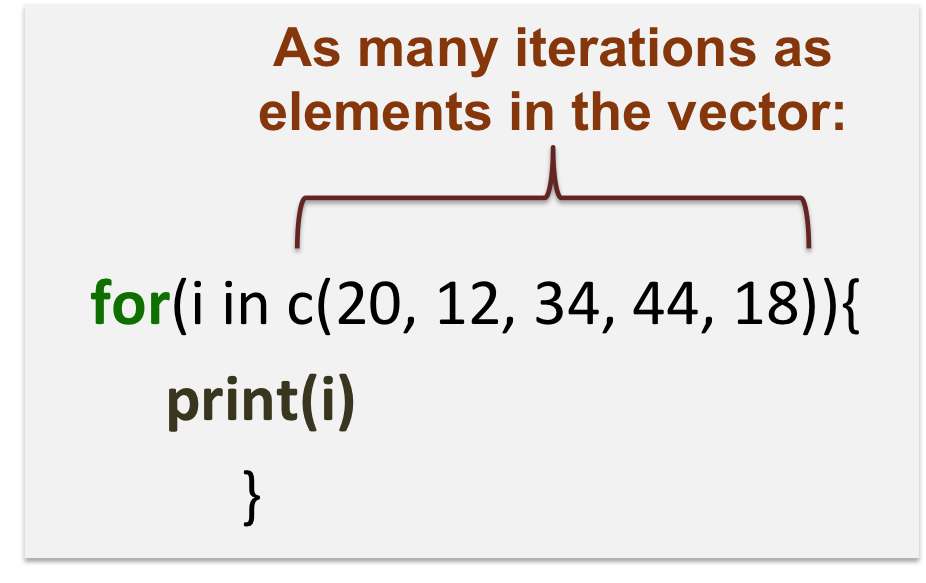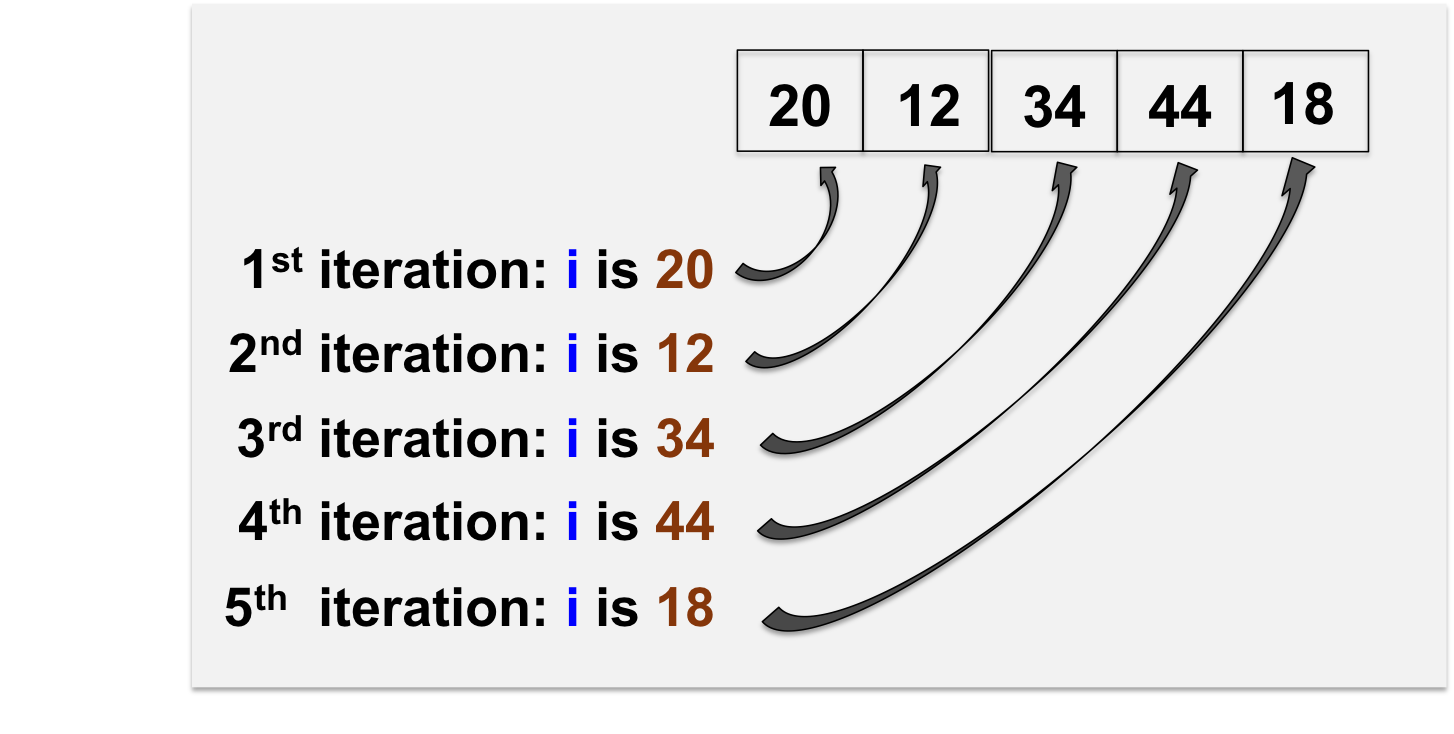# Part15 Repetitive execution

Loops are used to repeat a specific block of code.

Structure of the for loop:

``````for(i in vector_expression){
action_command
}``````

3 main elements:

• i is the loop variable: it is updated at each iteration.
• vector_expression: value attributed to i at each iteration (the number of iterations is the length of vector_expression).
• action_command: what action to take at each iteration.

Note the usage of curly brakets {} to start and end the loop!• Example:
``````for(i in 2:5){
# prints the value of i at each iteration
print(i)
# multiplies i by 2 at each iteration
y <- i*2
# prints the value of y at each iteration
print(y)
}``````
• Example of a for loop that iterates over a character vector:
``````# Character vector
myfruits <- c("apple", "pear", "grape")

# The for loop prints the current element j, and the number of characters of element j
for(j in myfruits){
print(j)
print(nchar(j))
}``````
• Example of a for loop that iterates over each row of a matrix, and prints the minimum value of that row :
``````# Matrix of 50 rows, 16 columns
mymat <- matrix(rnorm(800),
nrow=50)

# For loop over mymat rows
# 1:nrow(mymat): ranges from 1 to the number of rows in the matrix: 1, 2, 3, 4, ...., 48, 49, 50

for(i in 1:nrow(mymat)){
# prints the value of i at each iteration
print(i)
# prints the minimum value of the ith row of mymat at each iteration
print(min(mymat[i,]))
}``````
• If statement in For loop:

You can combine for loops and if statements:

``````# Matrix
mymat <- matrix(rnorm(800),
nrow=50)

# Loop over rows of mymat and print row if its median value is > 0
for(i in 1:nrow(mymat)){
# extract the current row
rowi <- mymat[i,]
# if median of row is > 0, print row
if(median(rowi) > 0){
print(rowi)
}
}``````

You can also have a look at this tutorial, to know more about loops.